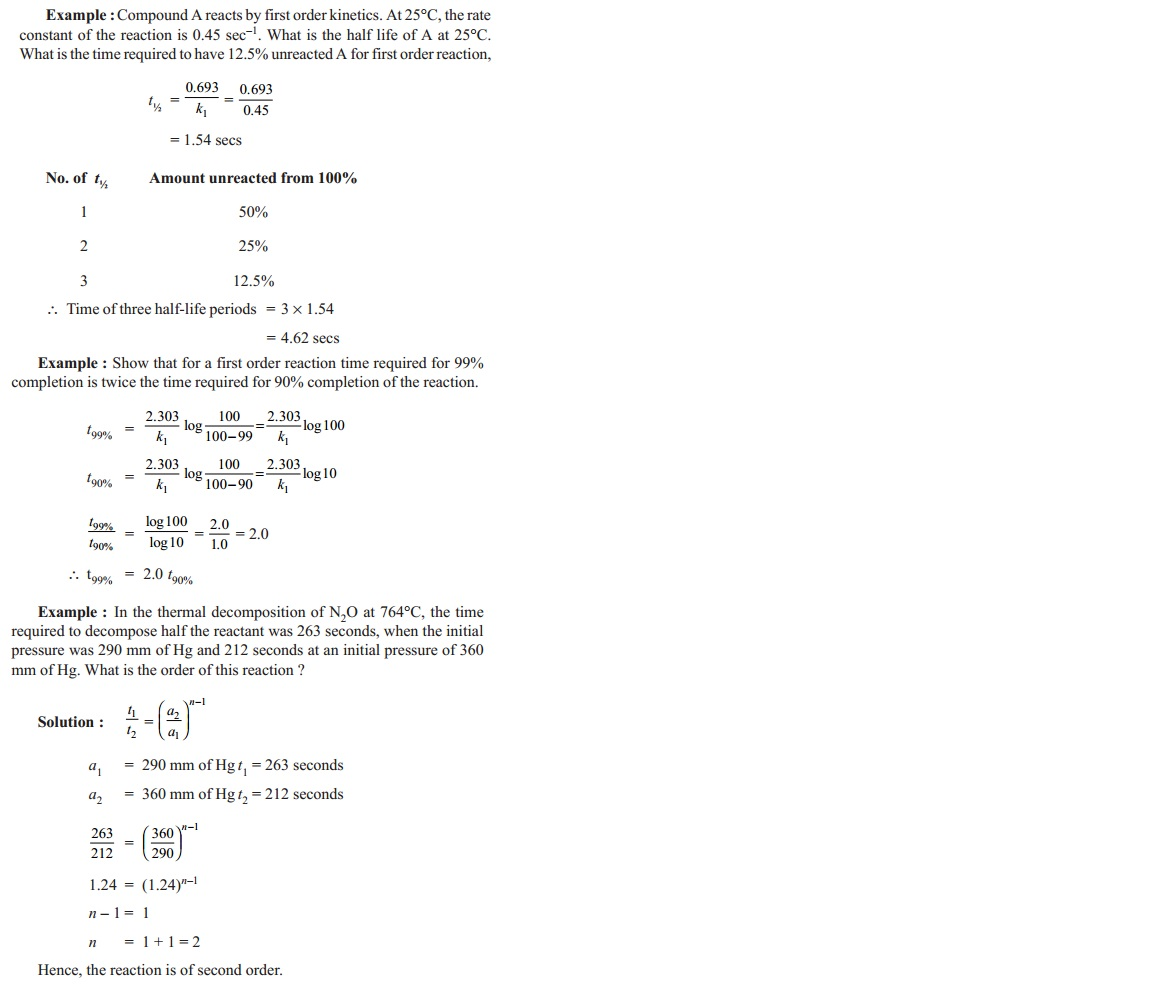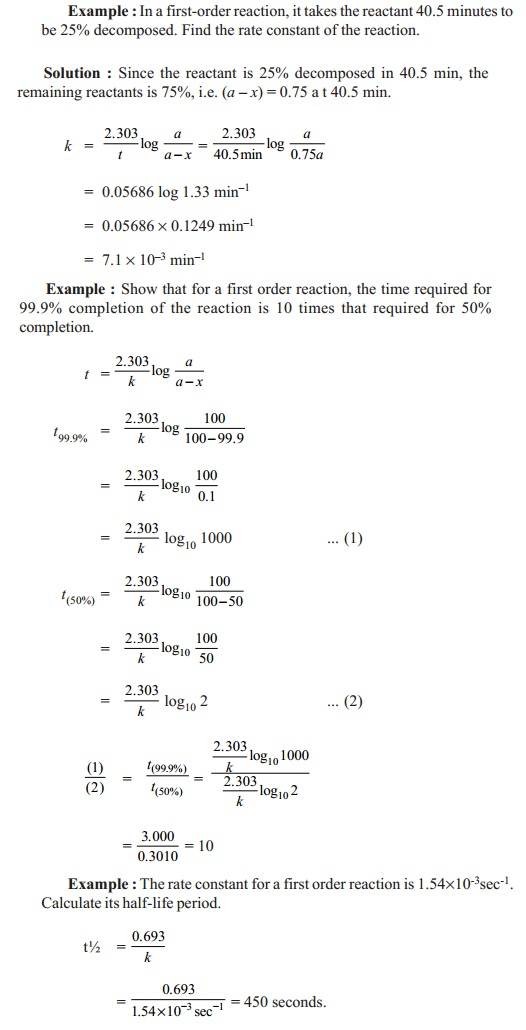Home | | Chemistry | Rate equation for first order reactions

# Rate equation for first order reactions

The reactions in which, the overall rate of the reaction is proportional to the first power of concentration of one of the reactants only are called as first order reactions. Consider the reaction

Rate equation for first order reactions

The reactions in which, the overall rate of the reaction is proportional to the first power of concentration of one of the reactants only are called as first order reactions. Consider the reaction

A  -- k1 -- > products

Rate of reaction  = -d[A] / dt  = = k [A]1.0

where k1 is the rate constant of the first order reaction.

At the beginning of the reaction, time ' t' = 0, let the concentration of A be ' a' mole.lit -1 . After the reaction has proceeded for some time 't', let the concentration of A that has reacted be x mole.lit-1 . The concentration of unreacted A remaining at time ' t' will be ( a - x) mole.lit-1 . The rate of the reaction will be dx/dt. For a first order reaction, rate  =  dx/dt  =  k1 (ax)   ��. (2)

upon integrating, equation 2 becomes,which is, -ln(a - x) = k  1t + c

c = integration constant

at time, t = 0, x = 0.

in equation 3,

- ln ( a - 0) = k1 0 +

C or C = -ln a.

Substituting C value in equation 3

Unit of k1 is sec-1

This equation is known as the first order rate constant equation.

This equation can be used for determining the rate constant of a first order reaction based on the experimental data of (a) and (a - x) at different periods of time 't'. Sometimes, the following expression is also used.

k1 = ( 2 303 / t2-t1 ) log (a-x1 / a-x2)

where x1 and x2 are the amounts reacted in t1 and t2 periods of time.

Characteristics of first order reaction

When the concentration of the reactant is increased by 'n' times, the rate of reaction is also increased by n times. That is, if the concentration of the reactant is doubled, the rate is doubled.

The unit of rate constant of a first order reaction is sec-1  or time-1 .

k1 = rate / ( a - x ) = mol.lit -1 sec- /  mol.lit -1 = sec-1

The time required to complete a definite fraction of reaction is independent of the initial concentration, of the reactant if t1/u is the time of one ' u'th fraction of reaction to take place then from equation 4,

x = a / u and

t1/u  = (2 303 / k1) log ( a/(a-a/u) )

t1/u  = (2 303 / k1) log ( u/(u-1) )

since k1 = rate constant, t1/u is independent of initial concentration 'a'.

Examples of first order reactions

92U238  -- -- >  90Th234        +       2He4

Decomposition of sulphuryl chloride in the gas phase proceed by first order kinetics.

SO2Cl2(g)     -- -- >   SO2(g)    +  Cl2(g)

Inversion of sucrose in acidic aqueous medium follows first order reaction.

C12H22O11 + H2O --H+ --- >   C6H12O6  (Glucose)  +   C6H12O6 (Fructose)Decomposition of nitrogen pentoxide in CCl4 medium also exhibits first order kinetics.

N2O5 --- > 2 NO2 + � O2

There are many other reactions that proceed by first order kinetics. We shall study some of the reactions that are experimentally followed by first order kinetic expressions including the parameters that change with concentration of the reactants or products which change with time of progress of the reaction.

Let us consider some of the first order reactions in detail :

1. Decomposition of nitrogen pentoxide in CCl4

N2O2 ---- k1 --- > 2NO2 + 1/2O2

At time t = 0, the volume of oxygen liberated is zero. Let Vt and V be the measured volumes of oxygen liberated after the reactant has reacted in 't' time and at completion (t = ). Initial concentration of N2O5 is proportional to total volume of oxygen liberated (i.e.,) (V).

(V-Vt) is proportional to undecomposed N2O5 at time ' t'.

K1 = (2.303 / t) log (  V /(V-Vt) )sec-12. Decomposition of H2O2 in aqueous solution

H2O2 --- Pt --- > H2O + � O2

The decomposition of H2O2 in aqueous medium in the presence of Pt catalyst follows a first order reaction. The progress of the reaction is followed by titrating equal volumes of the reaction mixture at regular time intervals against standard KMnO4 solution.

Since volume of KMnO4 used in the titration is a measure of concentration of undecomposed H2O2, volume of KMnO4 consumed at t = 0 is 'V o' which proportional to 'a', the initial concentration of H 2O2. Vt is proportional to unreacted H2O2 which is similarly (a - x ). Similarly (Vo-Vt) is proportional to ' x', the concentration of H2O2 reacted in time interval 't'. Vt is the volume of KMnO4 consumed after time ' t' of the reaction.

The first order rate constant ' k1' of the reaction is,

K1 = (2.303 / t) log (  V0 / Vt )sec-1Half life period 't'

Half life period, 't ', of a reaction is defined as the time required to reduce the concentration of a reactant to one half of its initial value. t values are calculated by using the integrated rate equation of any order of a reaction.

For first order reaction,

K1 = (2.303 / t) log (  a / (a-x) )sec-1

if amount reacted x = 2  then t= t1/2

t1/2 = (2.303 / k1) log (  a / (a-(a/2)) )

t1/2 = (2.303 / k1) log (2.0)

t1/2 = 0.693/ k1  secs

Thus half life period of a first order reaction is independent of the initial concentration of the reactant and also, inversely proportional to the rate constant of the reaction.Study Material, Lecturing Notes, Assignment, Reference, Wiki description explanation, brief detail
11th 12th std standard Class Organic Inorganic Physical Chemistry Higher secondary school College Notes : Rate equation for first order reactions |